## 缝合式C/SiC复合材料非线性本构关系及断裂行为研究$^{\bf 1)}$

*北京理工大学先进结构技术研究院, 北京 100081

## STUDY ON NONLINEAR CONSTITUTIVE RELATIONSHIP AND FRACTURE BEHAVIOR OF STITCHED C/SiC COMPOSITES$^{\bf 1)}$

Cao Mingyue*, Zhang Qi*, Wu Jianguo, Ge Jingran,*,2), Liang Jun,*,3)

*Institute of Advanced Structure Technology, Beijing Institute of Technology, Beijing 100081, China

Key Laboratory of Reliability and Environment Engineering Technology, Beijing Institute of Structure and Environment Engineering, Beijing 100076, China

 基金资助: 1)国家自然科学基金.  11802018国家自然科学基金.  111732002

Received: 2020-04-25   Accepted: 2020-06-1   Online: 2020-07-18C/SiC复合材料具有高比强度、高比模量和优良的热稳定性能等一系列优点, 广泛应用于航空航天领域中. 裂纹扩展进而引起的脆性断裂是其主要失效形式之一, 因而材料的断裂性能分析对材料的结构设计和应用有重要的指导意义. 本文开展了缝合式C/SiC复合材料简单力学试验和断裂试验, 研究了材料在不同载荷下的力学响应及断裂特征. 基于缝合式C/SiC复合材料简单力学试验, 建立了材料宏观非线性损伤本构方程, 并模拟了缝合式C/SiC复合材料单边切口梁和双悬臂梁的断裂行为. 本构方程采用简单函数描述了材料在复杂应力状态下的非线性应力-应变曲线, 并考虑了反向加载过程中造成的裂纹闭合. 基于商业有限元软件ABAQUS, 通过编写UMAT子程序实现非线性损伤本构方程, 采用单个单元验证了建立的本构方程的有效性. 在此基础上, 采用线弹性损伤本构和非线性损伤本构分别模拟了缝合式C/SiC复合材料单边切口梁和双悬臂梁的断裂行为. 采用非线性损伤本构方程模拟的力-位移曲线结果与试验结果更为吻合, 非线性损伤本构预测的失效载荷与试验失效载荷更为接近, 验证了所建立的非线性损伤本构方程的准确性, 为C/SiC复合材料断裂行为的研究提供了借鉴, 为缝合式C/SiC复合材料结构的设计和应用提供了理论基础.

Abstract

C/SiC composites have a series of advantages such as high specific strength, high specific modulus, excellent thermal stability, etc, being widely used in the aerospace field. Brittle fracture caused by crack propagation is one of its main failure forms. Therefore, the fracture performance analysis of the material has important guiding significance for the structural design and application of the material. Simple mechanical experiments and fracture experiments of stitched C/SiC composites are carried out, the mechanical response and fracture characteristics of the materials under different loads being studied in the paper. Based on simple mechanical experiments of stitched C/SiC composites, the macroscopic nonlinear damage constitutive equation is established, and the fracture behavior of stitched C/SiC composites with unilateral notched beam and double cantilever beam are simulated. The constitutive equation uses simple equations to describe the nonlinear stress-strain curve of the material under complex stress conditions, and considers the crack closure on the reverse loading process. Based on the commercial finite element software ABAQUS, the non-linear damage constitutive equations are realized by writing a UMAT subroutine. The validity of the established constitutive equation is verified by a single element. On this basis, the linear elastic damage constitutive model and the nonlinear damage constitutive model are used to simulate the fracture behavior of the stitched C/SiC composites with a single-side notched beam and a double cantilever beam, respectively. The force-displacement curves simulated by the nonlinear damage constitutive equation are more consistent with the test results, and the failure load predicted by the nonlinear damage constitutive are closer to the test failure load, which verifies the accuracy of the nonlinear damage constitutive equation established in this paper. The paper provides a reference for the study of the fracture behavior of C/SiC composites and provides a theoretical basis for the design and application of stitched C/SiC composites structures.

Keywords： stitched C/SiC composites ; nonlinear constitutive ; fracture ; damage

Cao Mingyue, Zhang Qi, Wu Jianguo, Ge Jingran, Liang Jun. STUDY ON NONLINEAR CONSTITUTIVE RELATIONSHIP AND FRACTURE BEHAVIOR OF STITCHED C/SiC COMPOSITES$^{\bf 1)}$. Chinese Journal of Theoretical and Applied Mechanics[J], 2020, 52(4): 1095-1105 DOI:10.6052/0459-1879-20-058

## 引言

C/SiC复合材料具有较高的比刚度、比强度及优良的高温性能, 在航空航天等领域有广阔的应用前景[1-3]. 国内外学者针对C/SiC复合材料的力学性能开展了广泛的试验研究[4-8], 裂纹扩展进而引起的脆性断裂是其主要失效形式之一[9-10]. C/SiC复合材料服役于航空航天飞行器的发动机、机翼等多个部位, 在材料的内部存在微小孔洞、微小裂纹等多种细观缺陷, 在受到外来载荷的作用时, 这些内部缺陷会进一步扩展形成宏观尺度上的裂纹, 表现为基体/纤维开裂、界面脱粘等, 最终可能会导致结构的破坏, 极大地影响了材料的使用寿命. 研究C/SiC复合材料的断裂行为对改善材料性能有重要的意义, 可以为结构设计和应用提供理论指导.

## 1 缝合式C/SiC复合材料力学试验

### 图1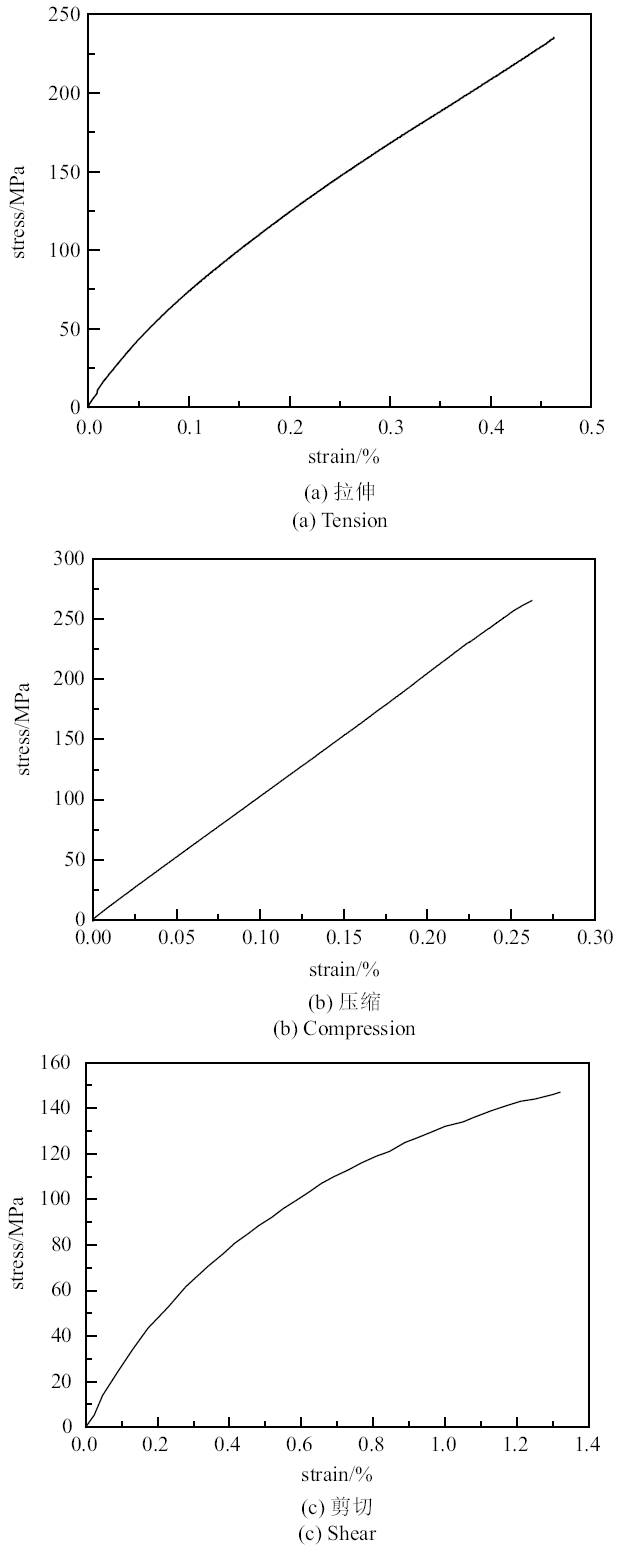Fig. 1   Experimental curve of basic mechanics

### 图2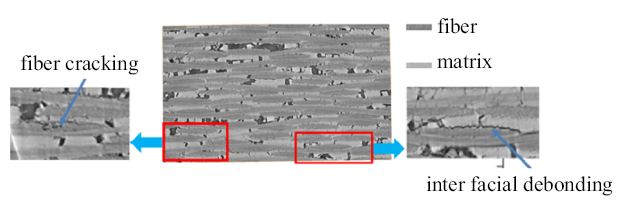Fig. 2   CT scan photos under tensile stress

### 图3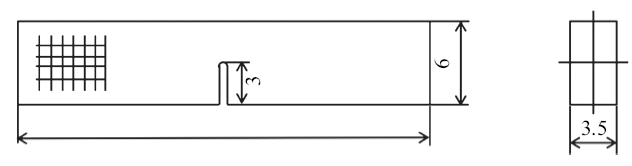Fig. 3   Single side notched beam (SENB) test piece

### 图4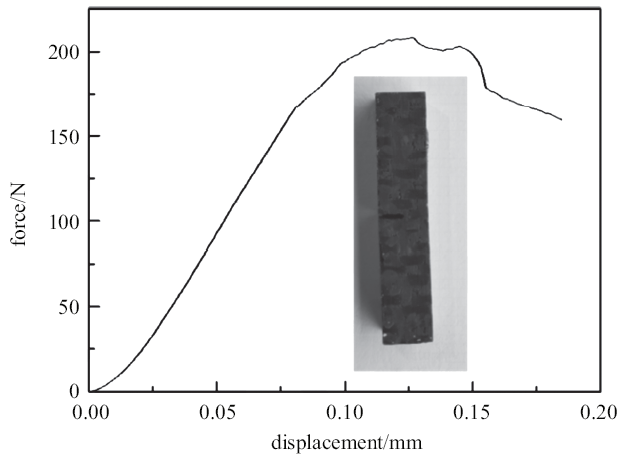Fig. 4   Force-displacement curve of single-sided notched beam

### 图5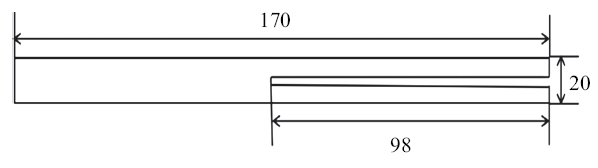Fig. 5   Double cantilever beam test piece

### 图6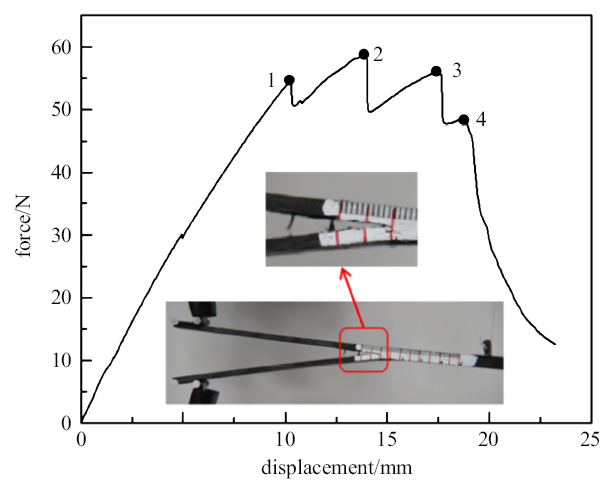Fig. 6   Force-displacement curve of double cantilever beam

## 2 宏观非线性损伤本构

### 图7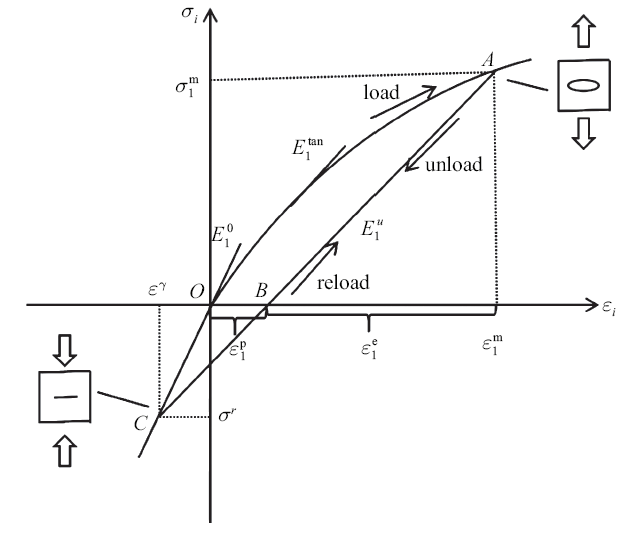### 2.1 非线性应力应变关系

\begin{eqnarray} \left\{ {\begin{array}{l} \varepsilon _1 \\ \varepsilon _2 \\ \varepsilon _6 \\ \end{array}} \right\}=\left[ {{\begin{array}{*{20}c} {1/E_1^0 } & {-v_{21}^0 /E_2^0 } & 0 \\ {-v_{12}^0 /E_1^0 } & {1/E_2^0 } & 0 \\ 0 & 0 & {1/G_{12}^0 } \\ \end{array} }} \right]\left\{ {\begin{array}{l} \sigma _1 \\ \sigma _2 \\ \sigma _6 \\ \end{array}} \right\}= S_0 \sigma \end{eqnarray}

\begin{eqnarray} \left\{ {\begin{array}{l} {\rm d}\sigma _1 \\ {\rm d}\sigma _2 \\ {\rm d}\sigma _6 \\ \end{array}} \right\}=\left[ {{\begin{array}{*{20}c} {E_1 E_1^0 E_2^0 /t} & {v_{21}^0 E_1^0 E_1 E_2 /t} & 0 \\ {v_{12}^0 E_2^0 E_1 E_2 /t} & {E_2 E_1^0 E_2^0 /t} & 0\\ 0 & 0 & {G_{12}} \\ \end{array} }} \right]\cdot \left\{ {\begin{array}{l} {\rm d}\varepsilon _1 \\ {\rm d}\varepsilon _2 \\ {\rm d}\varepsilon _6 \\ \end{array}} \right\}=C{\rm d}\varepsilon \end{eqnarray}

\begin{eqnarray} \left.\begin{array}{l} \sigma _i =A_1 \varepsilon _i +A_2 \varepsilon _i^2 +A_3 \varepsilon _i^3 +A_4 \varepsilon _i^4 +A_5 \varepsilon _i^5\\\qquad \left( {0\leqslant \varepsilon _i \leqslant \varepsilon _i^{\rm tb} ;i=1,2} \right) \\ \tau _{12} =B_1 \gamma _{12} +B_2 \gamma _{12}^2 +B_3 \gamma _{12}^3 +B_4 \gamma _{12}^4 +\\\qquad B_5 \gamma _{12}^5 \left( {\left| {\gamma _{12} } \right|\leqslant \gamma _{12}^{\rm b} } \right) \\ \end{array}\right\} \end{eqnarray}

\begin{eqnarray} \left.\begin{array}{l} E_i^{\tan } =A_1 +2A_2 \varepsilon _i +3A_3 \varepsilon _i^2 +4A_4\varepsilon _i^3 +5A_5 \varepsilon _i^4\\\qquad \left( {0\leqslant \varepsilon _i \leqslant \varepsilon _i^{\rm tb} ;i=1,2} \right) \\ G_{12}^{\tan } =B_1 +2B_2 \gamma _{12} +3B_3 \gamma _{12}^2 +4B_4 \gamma _{12}^3 +\\\qquad 5B_5 \gamma _{12}^4 \left( {\left| {\gamma _{12} } \right|\leqslant \gamma _{12}^{\rm b} } \right) \\ \end{array}\right\} \end{eqnarray}

\begin{eqnarray}\sigma _i =E_i^{\rm c} \varepsilon _i \left( {\varepsilon _i^{\rm cb} \leqslant \varepsilon _i \leqslant 0;i=1,2} \right)\end{eqnarray}

\begin{eqnarray}E_i^{\rm u} =A_6 +\frac{A_7 -A_6 }{\lt[1+\lt(\dfrac{\varepsilon _i^{\rm m} }{y_0 })^{e_0}]}\ \ (\varepsilon _i \leqslant \varepsilon _i^{\rm m} ,\sigma _i \geqslant \sigma ^{\rm r},i=1,2)\quad\end{eqnarray}

\begin{eqnarray}G_{12}^{\rm u} =B_6 +\frac{B_7 -B_6 }{1+\lt(\dfrac{\gamma _{12}^{\rm m} }{y_1 })^{e_1}}\left( {\left| {\gamma _{12} } \right|\leqslant \gamma _{12}^{\rm m} } \right)\end{eqnarray}

\begin{eqnarray}\frac{\sigma _1^2 }{X_{\rm t} X_{\rm c} }-\frac{\sigma _1 \sigma _2 }{X_{\rm t} X_{\rm c}}+\frac{\sigma _2^2 }{Y_{\rm t} Y_{\rm c} }+\frac{X_{\rm c} -X_{\rm t} }{X_{\rm t} X_{\rm c} }+\frac{Y_{\rm c} -Y_{\rm t}}{Y_{\rm t} Y_{\rm c} }+\frac{\tau _{12}^2 }{S^2}<1\quad\end{eqnarray}

Table 1  Parameters of stitched C/SiC composites constitutive model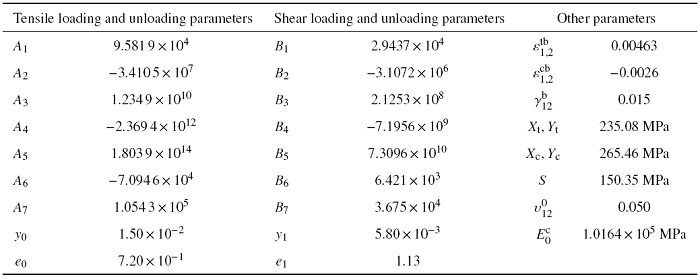### 2.2 数值实现

\begin{eqnarray}D=\frac{\partial \Delta \sigma }{\partial \Delta \varepsilon}\end{eqnarray}

\begin{eqnarray} \left\{ {\begin{array}{l} \Delta \sigma _1 \\ \Delta \sigma _2 \\ \Delta \sigma _6 \\ \end{array}} \right\}_{n+1} =\left[ {{\begin{array}{*{20}c} {C_{11} } & {C_{12} } & 0 \\ {C_{12} } & {C_{22} } & 0 \\ 0 & 0 & {C_{66} } \\ \end{array} }} \right]_{n+1} \left\{ {\begin{array}{l} \Delta \varepsilon _1 \\ \Delta \varepsilon _2 \\ \Delta \varepsilon _6 \\ \end{array}} \right\}_{n+1} \end{eqnarray}

UMAT程序中使用3个状态变量$\varepsilon _1^{\rm m}$, $\varepsilon _2^{\rm m}$和$\left|{\gamma _{12}^{\rm m} }\right|$分别记录材料在加卸载过程中所达到的最大拉伸应变和最大工程剪切应变. 通过比较应变分量与状态变量的大小判断材料的加卸载状态, 应变分量大于状态变量处于加载状态, 反之, 则处于卸载状态. 材料在反向压缩过程中, 压缩应力大于$\sigma ^{\rm r}$时, 保持为卸载模量, 反之, 模量变为$E_1^0$. 最后进行单元失效检查, 当Hoffman失效判据满足时, 刚度矩阵折减为接近于零的数值, 材料破坏. UMAT具体流程如下所示:

(1)读取$n+1$步的应变张量 $\varepsilon _{n+1} =\varepsilon _n +\Delta \varepsilon _{n+1}$;

(2)判断加卸载状态 $\varepsilon _{i,n+1} \Leftrightarrow \varepsilon ^{\rm m}_i$, $\sigma _{i,n} \Leftrightarrow \sigma ^{\rm r}$;

(3)计算损伤模量 $E_{1}$, $E_{2}$, $G_{12}$;

(4)计算名义应力张量 $\left\{ {\sigma _i } \right\}_{n+1} =\left\{ {\sigma _i } \right\}_n +\left\{ {C_{ij} } \right\}_{n+1} \left\{ {\Delta \varepsilon _j } \right\}_{n+1}$;

(5)计算雅可比矩阵 $D=\dfrac{\partial \Delta \sigma}{\partial \Delta \varepsilon}$;

(6)失效判断.

### 图8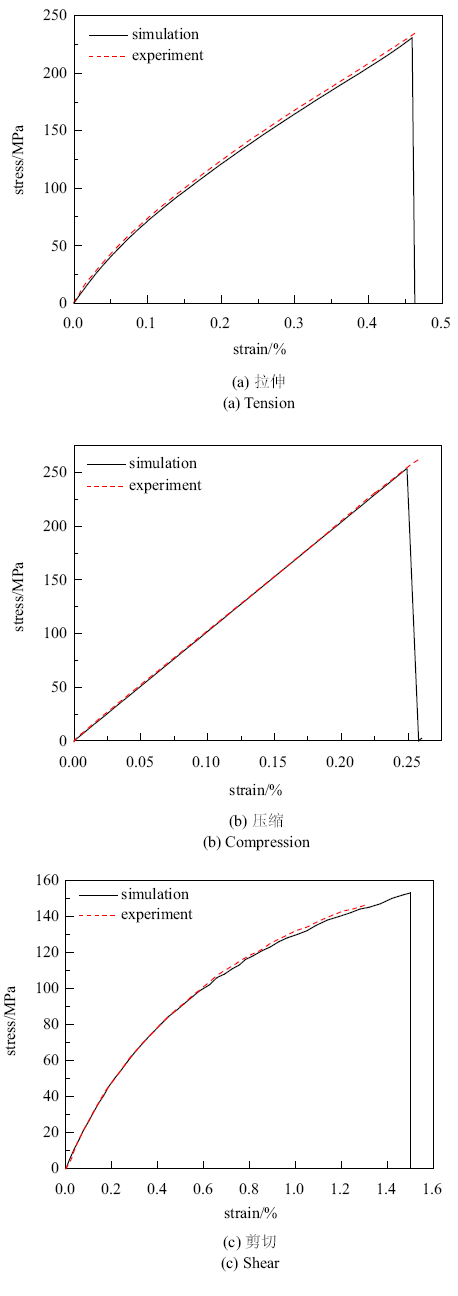### 图9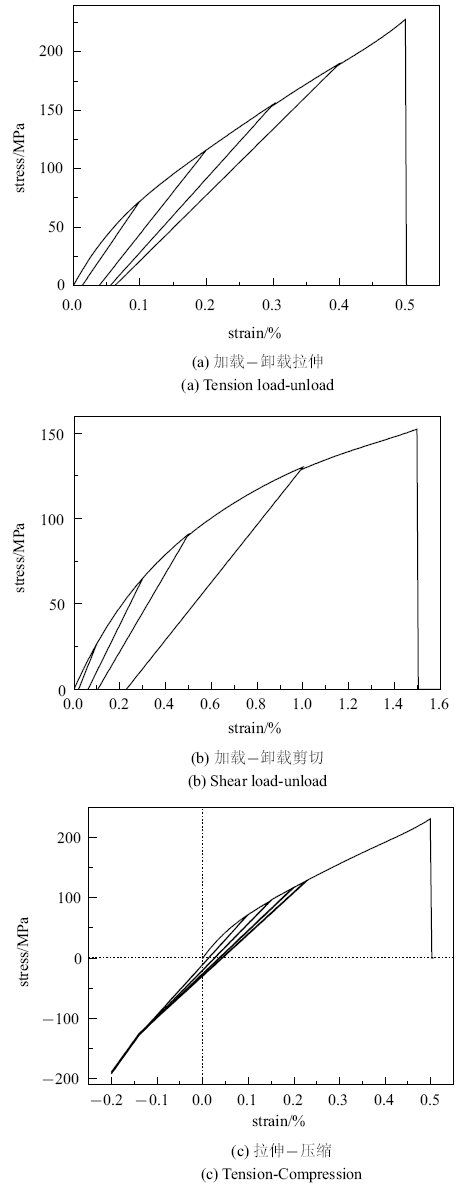Fig. 9   Stress-strain curve of materials under complex stress

## 3 缝合式C/SiC复合材料断裂行为模拟

### 图10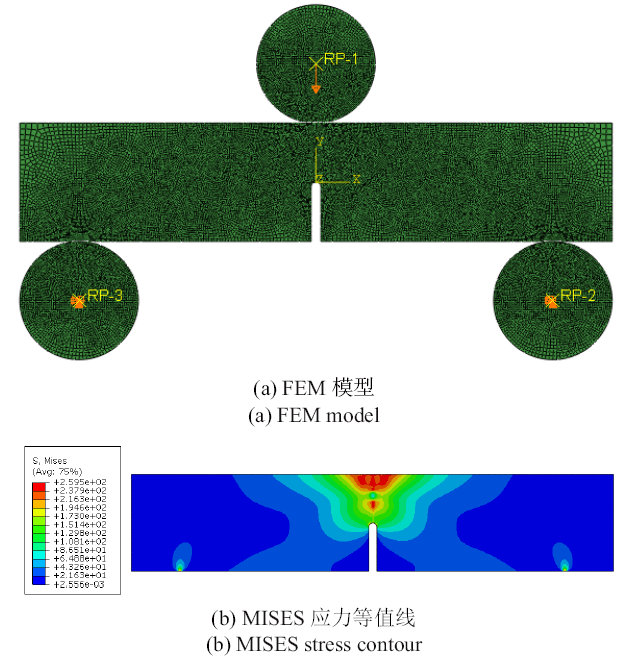Fig. 10   Single-sided notched beam model

### 图11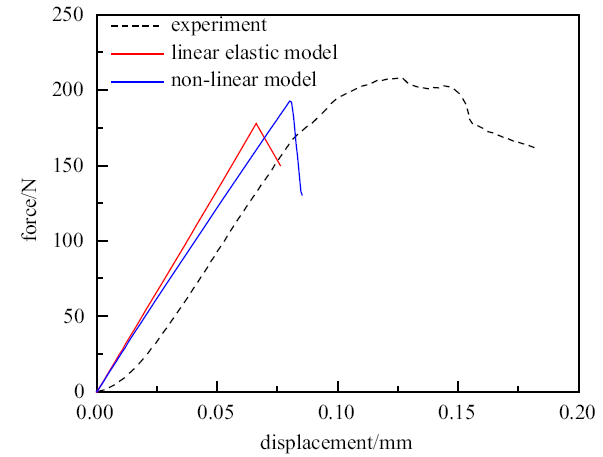Fig. 11   Comparison of simulation and experimentalresults of single-sided notched beam

Table 2  Comparison of finite element and experimental results of single-sided notched beam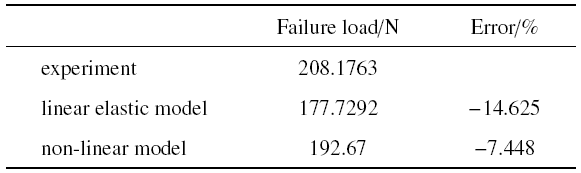### 图12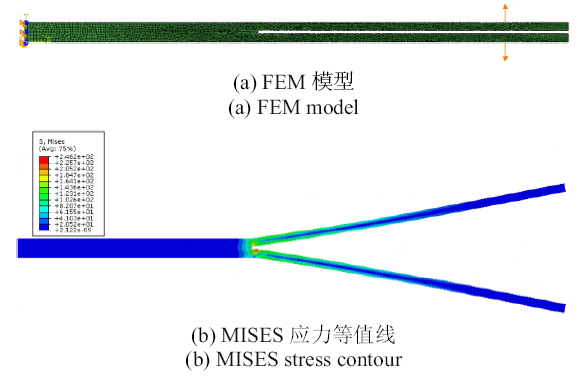Fig. 12   Double cantilever beam model

### 图13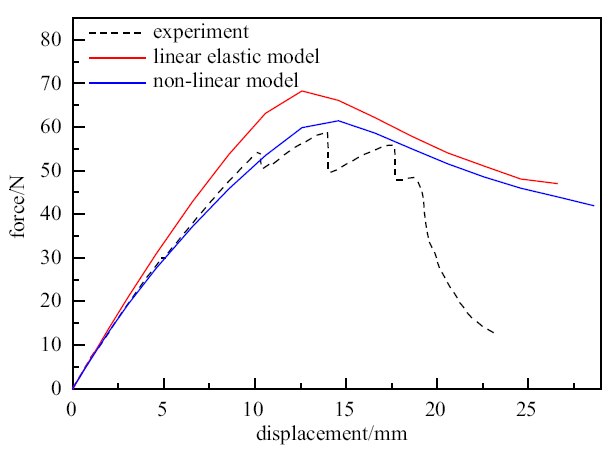Fig. 13   Comparison of simulation and test results ofdouble cantilever beam

Table 3  Comparison of finite element and experimentalresults of double cantilever beams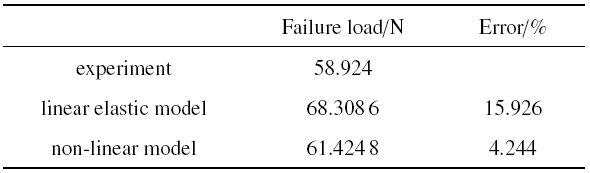## 4 结论

(1)本文依据简单力学试验及CT扫描结果, 为缝合式C/SiC复合材料建立了宏观非线性本构方程. 本构方程中参数可以通过数量较少的简单加载及加卸载力学试验获得. 本构方程可以区别加载、卸载、重加载三种状态, 并考虑了反向加载过程中造成的裂纹闭合, 可以描述缝合式C/SiC复合材料在复杂应力状态下的力学行为.

(2)在ABAQUS中, 结合UMAT模拟缝合式C/SiC复合材料单边切口梁和双悬臂梁的断裂行为. 与线弹性本构对比, 非线性本构模拟结果与试验更加吻合. 说明本文建立的非线性本构可以较好地预测缝合式C/SiC复合材料单边切口梁和双悬臂梁的断裂行为.

(3)本文基于简单力学试验建立了缝合式C/SiC复合材料的宏观唯象本构方程, 未区分纤维、基体和缺陷, 在后续工作中将建立复合材料的细观模型, 把孔隙作为单独的因素考虑, 结合现有宏观模型, 实现对C/SiC复合材料的多尺度分析.

## 参考文献 原文顺序 文献年度倒序 文中引用次数倒序 被引期刊影响因子

( Chen Qian, Zhang Hanzhe, Wu Qin, et al.

Numerical study on hydrodynamic and structural strength characteristics of composite hydrofoil

Chinese Journal of Theoretical and Applied Mechanics, 2019,51(5):1350-1362 (in Chinese))

( Liu Gen, Zhang Wei.

Research on nonlinear vibration response of composite cantilever plate in subsonic airflow

Chinese Journal of Theoretical and Applied Mechanics, 2019,51(3):912-921 (in Chinese))

( Li Wei, Fang Guodong, Li Weijie, et al.

Numerical simulation of micro-ablation behavior of carbon fiber reinforced composites

Chinese Journal of Theoretical and Applied Mechanics, 2019,51(3):835-844 (in Chinese))

Nozawa T, Ozawa K, Choi YB, et al.

Determination and prediction of axial/off-axial mechanical properties of SiC/SiC composites

Fusion Engineering and Design, 2012,87(5-6):803-807

URL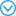A SiC/SiC composite is a candidate material for a demonstration fusion power reactor (DEMO). Identifying the inherent anisotropy of composites is required to predict axial/off-axial mechanical properties for various failure modes. This study evaluated crack propagation behavior by the various modes to provide strength anisotropy maps and we discussed a method to analytically predict this trend. The strength anisotropy maps identified for various fabric orientations clearly indicate that the composites failed in the mixed modes. Specifically, due to the axial anisotropy, five individual mode strengths such as tensile/compressive strengths in the axial/transverse directions, respectively, as well as the in-plane shear strength, are identified to become essential input parameters. With the analytical criterion based on the Tsai-Wu model, the strength anisotropy could satisfactorily be described. (C) 2012 Elsevier B.V.

Li J, Jiao G, Wang BD, et al.

Damage characteristics and constitutive modeling of the 2D C/Si C composite: Part I-Experiment and analysis

Chinese Journal of Aeronautics, 2014,27(6):1586-1597

URLThis paper reports an experimental investigation on the macroscopic mechanical behaviors and damage mechanisms of the plain-woven (2D) C/SiC composite under in-plane on-and off-axis loading conditions. Specimens with 15 degrees, 30 degrees, and 45 degrees off-axis angles were prepared and tested under monotonic and incremental cyclic tension and compression loads. The obtained results were compared with those of uniaxial tension, compression, and shear specimens. The relationships between the damage modes and the stress state were analyzed based on scanning electronic microscopy (SEM) observations and acoustic emission (AE) data. The test results reveal the remarkable axial anisotropy and unilateral behavior of the material. The off-axis tension test results show that the material is fiber-dominant and the evolution rate of damage and inelastic strain is accelerated under the corresponding combined biaxial tension and shear loads. Due to the damage impediment effect of compression stress, compression specimens show higher mechanical properties and lower damage evolution rates than tension specimens with the same off-axis angle. Under cyclic tension-compression loadings, both on-axis and off-axis specimens exhibit progressive damage deactivation behaviors in the compression range, but with different deactivation rates. (C) 2014 Production and hosting by Elsevier Ltd. on behalf of CSAA & BUAA.

Dassios KG, Kostopoulos V, Steen M.

Intrinsic parameters in the fracture of carbon/carbon composites

Composites Science and Technology, 2005,65(6):883-897

URL## Abstract

The R-curve, bridging stress profile and the corresponding bridging law of C/C woven composites were calculated for two different specimen configurations: compact tension (CT) and double-edge-notch (DEN). Monotonic tensile loading as well as cyclic loading was performed on CT specimens and the R-curve was evaluated from the change of specimen compliance in the presence and absence of the bridging zone by quantifying the total damage in the material through an effective crack length approach. The bridging stresses and laws for the DEN specimens tested under monotonic tension were calculated directly through the elastic correction of the measured displacement. The comparison of the R-curves and bridging laws between the two configurations was performed after identification of a common damage extent measure, the local crack opening displacement. The rising part of the R-curve was found similar for the two configurations, with the mean initiation and plateau values being R0 ∼ 1.3 and R ∼ 9.5 kJ/m2, respectively. In contrast, the bridging laws evaluated for the two configurations were in disagreement. It was concluded that the investigated material, although characterised as Class-III in the literature, exhibits crack growth and bridging, however of a Small rather of a large scale and that the calculated R-curve can serve as an intrinsic parameter that characterises the fracture behaviour of the material independently of configuration, and – thus – independently of dimensions and geometry.

Xie D, Salvi A, Waas A, et al.

Discrete cohesive zone model to simulate static fracture in carbon fiber composites

Journal of Composite Materials, 2005,40(22):2025-2046

Liu MS, Li YL, Xu F, et al.

Dynamic compressive mechanical properties and a new constitutive model of 2D-C/SiC composites

Materials Science and Engineering, 2008,489(1-2):120-126

( Lu Guangda, Chen Jianbing.

Cracking simulation based on a nonlocal macro-meso-scale damage model

Chinese Journal of Theoretical and Applied Mechanics, 2020,52(3):749-762 (in Chinese))

( Wang Bo, Zhang Yangbo, Zuo Hong, et al.

Study on the inflfluence of compressive stress on the compression shear crack propagation

Chinese Journal of Theoretical and Applied Mechanics, 2019,51(3):845-851 (in Chinese))

Finite element solution of the fiber/matrix interface crack problem: Convergence properties and mode mixity of the Virtual Crack Closure Technique

Finite Elements in Analysis and Design, 2019,167:103332

Shokrieh MM, Rajabpour-Shirazi H, Heidari-Rarani M.

Simulation of mode I delamination propagation in multidirectional composites with R-curve effects using VCCT method

Computational Materials Science, 2012,65:66-73

URLIn this investigation, a finite element formulation for Timoshenko beam element with only displacement degrees of freedom is first addressed for the laminated composite beams. The resulting continuous isoparametric quadrilateral element is simple to formulate and efficient through the convergence with coarse meshes along the crack tip. Afterwards, a finite element procedure is proposed for the simulation of mode I delamination growth in symmetric multidirectional double cantilever beam (DCB) specimens based on the fracture mechanics using the above-mentioned element. To take into account R-curve effects in DCB specimens, a variable strain energy release rate is utilized instead of constant initiation fracture toughness. The strain energy release rate is computed using virtual crack closure technique (VCCT) method. The results of the finite element simulation agree well with the experimental data available in the literature. It confirms that the proposed approach is reliable and feasible for modeling of mode I delamination growth in laminated composites with large-scale fiber bridging. (c) 2012 Elsevier B.V.

Liu PF, Hou SJ, Chu JK, et al.

Finite element analysis of postbuckling and delamination of composite laminates using virtual crack closure technique

Composite Structures, 2011,93(6):1549-1560

URLThe two-dimensional and three-dimensional parametric finite element analysis (FEA) of composite flat laminates with two through-the-width delamination types: 0(4)/(+/-theta)(6)//0(4) and 0(4)//(+/-theta)(6)//0(4) (theta = 0 degrees, 45 degrees, and "//" denotes the delaminated interface) under compressive load are performed to explore the effects of multiple delaminations on the postbuckling properties. The virtual crack closure technique which is employed to calculate the energy release rate (ERR) for crack propagation is used to deal with the delamination growth. Three typical failure criteria: B-K law, Reeder law and Power law are comparatively studied for predicting the crack propagation. Effects of different mesh sizes and pre-existing crack length on the delamination growth and postbuckling properties of composite laminates are discussed. Interaction between the delamination growth mechanisms for multiple cracks for 0(4)//(+/-theta)(6)//0(4) composite laminates is also investigated. Numerical results using FEA are also compared with those by existing models and experiments. (C) 2010 Elsevier Ltd.

Aymerich F, Dore F, Priolo P.

Prediction of impact-induced delamination in cross-ply composite laminates using cohesive interface elements

Composites Science and Technology, 2008,68(12):2383-2390

URL## Abstract

The paper investigates the potential of cohesive interface elements for damage prediction in laminates subjected to low-velocity impact. FE models with interface elements adopting a bilinear cohesive law were first calibrated and validated by simulation of standard fracture toughness tests and then employed to model the impact response of cross-ply graphite/epoxy laminated plates.

The developed model provided a correct simulation of the impact response of laminates in a wide range of energy values and successfully predicted size, shape and location of main damage mechanisms. The results of the analyses also pointed out the importance of employing a damage criterion capable of accounting for the constraining effect of out-of-plane compression on the initiation of the decohesion phase.

Harper PW, Hallett SR.

A fatigue degradation law for cohesive interface elements-Development and application to composite materials

International Journal of Fatigue, 2010,32(11):1774-1787

URL## Abstract

A cohesive zone interface element degradation law is presented for analyzing delamination crack propagation under cyclic loading. Development of the law is based on a detailed study of the numerical cohesive zone and the extraction of strain energy release rate from this zone, enabling a direct link with experimental Paris Law data. The law is implemented using three-dimensional interface elements within the explicit finite element code LS-Dyna. Validation is presented by way of application to composite material fatigue fracture toughness tests; Double Cantilever Beam for Mode I, End Notch Flexure for mode II and Mixed Mode Bending for the mixed mode case. In all cases good agreement with experimental data available in the open literature and/or theoretical solutions was obtained.

( Wang Zhikai, Chen Zhipeng, Yang Nana, et al.

Study on the effect of initial defects on the mechanical properties of composite laminates

Journal of Northwestern Polytechnical University, 2019,37(4):730-736 (in Chinese))

Fracture analysis of composites by time independent moving-crack orthotropic XFEM

International Journal of Mechanical Sciences, 2012,54(1):20-37

URLTime-independent orthotropic enrichment functions are introduced for dynamic propagation analysis of moving cracks in composites by the extended finite element method (XFEM). The proposed enrichment functions are derived from the analytical solutions for a moving/propagating crack in orthotropic media, and can be considered as a new extension to the available XFEM techniques for dynamic analysis of stationary and moving cracks in orthotropic materials. They are included within the framework of partition of unity and XFEM to enhance the accuracy of basic FEM solution near a moving crack tip in orthotropic media. The method allows for analysis of the whole crack propagation pattern on an unaltered finite element mesh, which is independently defined from the existence of any predefined crack or its propagation path. A combination of dynamic crack initiation toughness and crack orientation along the maximum circumferential stress is used to design a relatively simple and efficient formulation. Dynamic stress intensity factors (DSIFs) are evaluated by means of the domain separation integral method and the dynamic energy release rate. The time dependent XFEM equations are constructed by discretizing the standard weak formulation of the governing elastodynamics equation. They are solved by the unconditionally stable Newmark time integration scheme. A number of benchmark and test problems are simulated and the results are compared with the available reference results to illustrate the accuracy and efficiency of the proposed scheme. (C) 2011 Elsevier Ltd.

Baydoun M, Fries T P.

Crack propagation criteria in three dimensions using the XFEM and an explicit-implicit crack description

International Journal of Fracture, 2012,178(1-2):51-70

URLThis paper studies propagation criteria in three-dimensional fracture mechanics within the extended finite element framework (XFEM). The crack in this paper is described by a hybrid explicit-implicit approach as proposed in Fries and Baydoun (Int J Numer Methods Eng, 2011). In this approach, the crack update is realized based on an explicit crack surface mesh which allows an investigation of different propagation criteria. In contrast, for the computation of the displacements, stresses and strains by means of the XFEM, an implicit description by level set functions is employed. The maximum circumferential stress criterion, the maximum strain energy release rate criterion, the minimal strain energy density criterion and the material forces criterion are realized. The propagation paths from different criteria are studied and compared for asymmetric bending, torsion, and combined bending and torsion test cases. It is found that the maximum strain energy release rate and maximum circumferential stress criterion show the most favorable results.

Chen C, Cai HY, Li JJ, et al.

One-dimensional extended FEM based approach for predicting the tensile behavior of SHCC-FRP composites

Engineering Fracture Mechanics, 2020,225:106775

Ma X, Bian K, Liu H, et al.

Numerical and experimental investigation of the interface properties and failure strength of CFRP T-Stiffeners subjected to pull-off load

Materials & Design, 2020,185:108231

I Ud Din, Hao P, Franz G, et al.

Elastoplastic CDM model based on Puck's theory for the prediction of mechanical behavior of Fiber Reinforced Polymer (FRP) composites

Composite Structures, 2018(201):291-302

Xie J, Fang G, Chen Z, et al.

An anisotropic elastoplastic damage constitutive model for 3D needled C/C-SiC composites

Composite Structures, 2017,176:164-177

Li J, Jiao G, Wang B, et al.

Damage characteristics and constitutive modeling of the 2D C/SiC composite: Part II-Material model and numerical implementation

Chinese Journal of Aeronautics, 2015,28(1):314-326

URLIn this work, a macroscopic non-linear constitutive model accounting for damage, inelastic strain and unilateral behavior is proposed for the 2D plain-woven C/SiC composite. A set of scalar damage variables and a new thermodynamic potential expression are introduced in the framework of continuum damage mechanics. In the deduced constitutive equations, the material's progressive damage deactivation behavior during the compression loading is described by a continuous function, and different deactivation rates under uniaxial and biaxial compression loadings are also considered. In damage evolution laws, the coupling effect among the damage modes and impediment effect of compression stress on the development of shear damage in different plane stress states are taken into account. Besides, the general plasticity theory is applied to describing the evolution of inelastic strain in tension and/or shear stress state. The Tsai-Wu failure criterion is adopted for strength analysis. Additionally, the material model is implemented as a user-defined material subroutine (UMAT) and linked to the ABAQUS finite element software, and its performance is demonstrated through several numerical examples.

Wei L, Zhu W, Yu Z, et al.

A new three-dimensional progressive damage model for fiber-reinforced polymer laminates and its applications to large open-hole panels

Composite Science and Technology, 2019,182:107757

( Yang Zhengmao, Liu Hui, Yang Junjie.

Damage constitutive model forthermal shocked-ceramic matrix composite

Chinese Journal of Theoretical and Applied Mechanics, 2019,51(6):1797-1809 (in Chinese))

( Zhang Genxi, Zhang Peiwei, Li Yanbin, et al.

Acta Materiae Compositae Sinica, 2019,36(12):2894-2901 (in Chinese))

( Li Yan, Cui Hong, Wang Bin, et al.

Influence of densification process on the properties of thick-wall needle-punched C/C composites

Acta Materiae Compositae Sinica, 2017,34(10):2337-2343 (in Chinese))

URLBased on the fundamental mechanical tests of 2D woven C/SiC composite material, a macroscopic orthotropic non-linear damage constitutive model was established. In this model, the detectable strains were chosen as variables, and functions in simple form were used to demonstrate the stress-strain relationship of the material under damage evolution caused by uniaxial tension and shear loads, and besides the rule of stiffness change in unloading state respectively. Meanwhile, the unilateral effect and damage-deactivation behavior were also considered. The constitutive model was implemented into UMAT in ABAQUS finite element software, and it could describe the material’s non-linear and linear property of stress-strain relationship in loading and unloading state respectively, and also its load history. Through modeling the open hole plates in tension, strain distribution besides the hole agrees well with the experiment result, which proves the availability of the proposed constitutive model.

( Li Jun, Jiao Guiqiong, Wang Bo, et al.

Nonlinear damage constitutive model of 2D braided C/SiC composites and its application

Acta Materiae Compositae Sinica, 2013,30(1):165-171 (in Chinese))

URLBased on the fundamental mechanical tests of 2D woven C/SiC composite material, a macroscopic orthotropic non-linear damage constitutive model was established. In this model, the detectable strains were chosen as variables, and functions in simple form were used to demonstrate the stress-strain relationship of the material under damage evolution caused by uniaxial tension and shear loads, and besides the rule of stiffness change in unloading state respectively. Meanwhile, the unilateral effect and damage-deactivation behavior were also considered. The constitutive model was implemented into UMAT in ABAQUS finite element software, and it could describe the material’s non-linear and linear property of stress-strain relationship in loading and unloading state respectively, and also its load history. Through modeling the open hole plates in tension, strain distribution besides the hole agrees well with the experiment result, which proves the availability of the proposed constitutive model.

Mccarthy CT, O'Higgins RM, Frizzell RM.

A cubic spline implementation of non-linear shear behaviour in three-dimensional progressive damage models for composite laminates

Composite Structures, 2010,92(1):173-181

URL## Abstract

An experimental study was carried out to characterise the constitutive response of carbon fibre-reinforced epoxy laminates. While maintaining essentially linear behaviour in the fibre and transverse directions, this material displays significant non-linear shear stress–strain behaviour to rupture. It is shown that the well known Hahn-Tsai non-linear shear model does not provide an acceptable fit for the strain range examined and so a novel approach was derived where a cubic spline interpolation method was used to capture the non-linear shear behaviour. The well known ply discount model, based on Hashin’s failure criteria, was also used to predict fibre and transverse matrix damage in the laminates. The spline approach is coupled with maximum strain failure criteria to predict the response in the in-plane and out-of-plane shear directions. The material Jacobian matrix is fully defined, thus allowing a full implicit material model to be implemented. Hence, the model is suitable for both implicit and explicit finite element codes. It is shown that the model accurately predicts the response of the material for load cases in which shear stresses dominate. The performance of the model is demonstrated by considering a number of laminate configurations and failure of an open-hole tension specimen.

Elias N Eliopoulos, Theodore P Philippidis.

A progressive damage simulation algorithm for GFRP composites under cyclic loading. Part I: Material constitutive model

Composite Science and Technology, 2001,71:742-749

( Huang Wenchao, Zhu Zhaoxi.

Failure analysis of outer tension of aircraft composite long truss

Journal of Mechanical Strength, 2019,41(3):248-252 (in Chinese))

Schellekens JCJ, Borst RD.

The use of the Hoffman yield criterion in finite element analysis of anisotropic composites

Computers & Structures, 1990,37(6):1087-1096

/

 〈〉